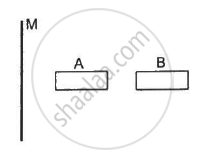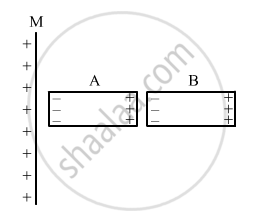Department of Pre-University Education, KarnatakaPUC Karnataka Science Class 12
Share

# A Large Non-conducting Sheet M is Given a Uniform Charge Density. Two Uncharged Small Metal Rods a and B Are Placed Near the Sheet as Shown in the Following Figure . - Physics

ConceptUniformly Charged Infinite Plane Sheet and Uniformly Charged Thin Spherical Shell (Field Inside and Outside)

#### Question

A large non-conducting sheet M is given a uniform charge density. Two uncharged small metal rods A and B are placed near the sheet as shown in the following  figure.

(a) M attracts A.
(b) M attracts B.
(c) A attracts B.
(d) B attracts A.#### Solution

(a) M attracts A.
(b) M attracts B.
(c) A attracts B.
(d) B attracts A.Since the non-conducting sheet M is given a uniform charge, it induces a charge in the metal rod A, which further induces a charge in the metal rod B, as shown in the figure. Hence, all the options are correct.

Is there an error in this question or solution?

#### APPEARS IN

HC Verma Solution for Concepts of Physics - Vol. 2 (2018 to Current)
Chapter 8: Gauss’s Law
MCQ | Q: 3 | Page no. 140

#### Video TutorialsVIEW ALL 

Solution A Large Non-conducting Sheet M is Given a Uniform Charge Density. Two Uncharged Small Metal Rods a and B Are Placed Near the Sheet as Shown in the Following Figure . Concept: Uniformly Charged Infinite Plane Sheet and Uniformly Charged Thin Spherical Shell (Field Inside and Outside).
S